# Recursion Recursion Recursion is the name given for

• Slides: 22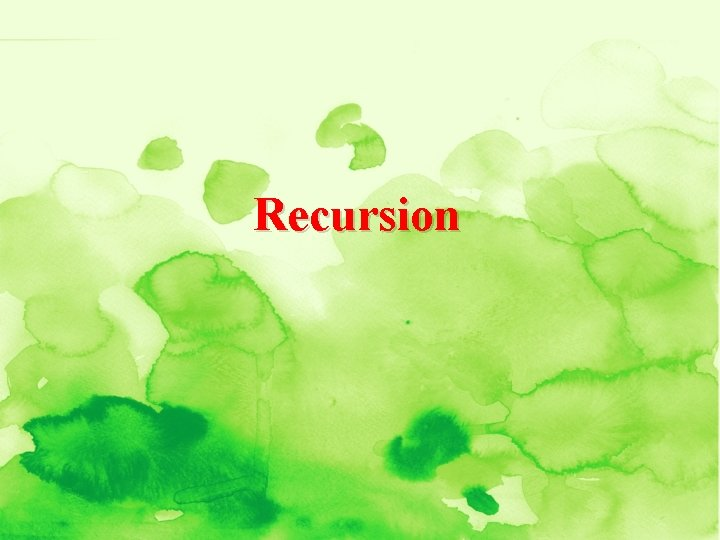Recursion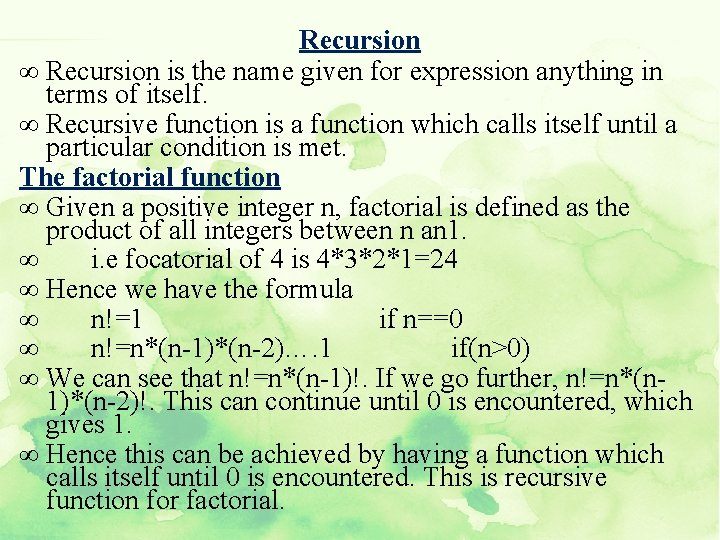Recursion ∞ Recursion is the name given for expression anything in terms of itself. ∞ Recursive function is a function which calls itself until a particular condition is met. The factorial function ∞ Given a positive integer n, factorial is defined as the product of all integers between n an 1. ∞ i. e focatorial of 4 is 4*3*2*1=24 ∞ Hence we have the formula ∞ n!=1 if n==0 ∞ n!=n*(n-1)*(n-2)…. 1 if(n>0) ∞ We can see that n!=n*(n-1)!. If we go further, n!=n*(n 1)*(n-2)!. This can continue until 0 is encountered, which gives 1. ∞ Hence this can be achieved by having a function which calls itself until 0 is encountered. This is recursive function for factorial.Ex: 5!=5* 4! 120 4 * 3! 24 3 * 2! 6 2 * 1! 2 1 * 0! 1 1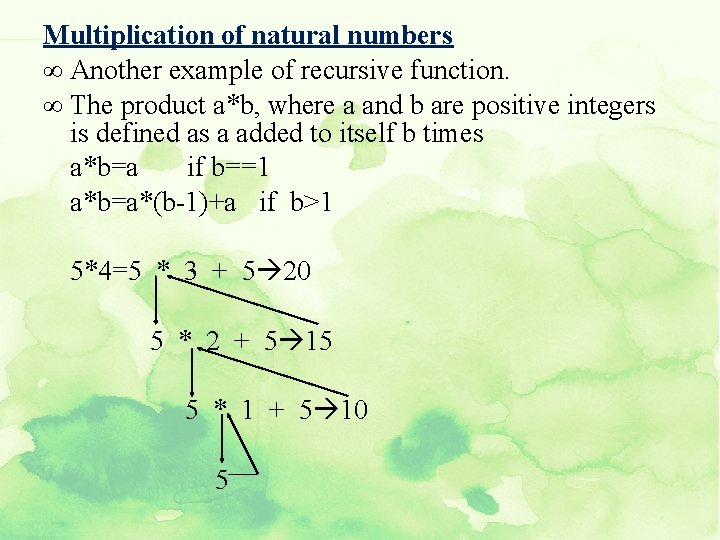Multiplication of natural numbers ∞ Another example of recursive function. ∞ The product a*b, where a and b are positive integers is defined as a added to itself b times a*b=a if b==1 a*b=a*(b-1)+a if b>1 5*4=5 * 3 + 5 20 5 * 2 + 5 15 5 * 1 + 5 10 5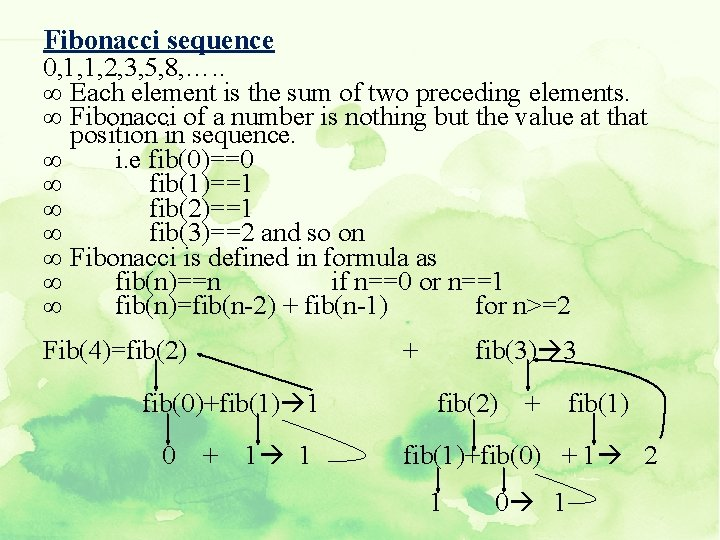Fibonacci sequence 0, 1, 1, 2, 3, 5, 8, …. . ∞ Each element is the sum of two preceding elements. ∞ Fibonacci of a number is nothing but the value at that position in sequence. ∞ i. e fib(0)==0 ∞ fib(1)==1 ∞ fib(2)==1 ∞ fib(3)==2 and so on ∞ Fibonacci is defined in formula as ∞ fib(n)==n if n==0 or n==1 ∞ fib(n)=fib(n-2) + fib(n-1) for n>=2 Fib(4)=fib(2) + fib(0)+fib(1) 1 0 + 1 1 fib(3) 3 fib(2) + fib(1)+fib(0) + 1 2 1 0 1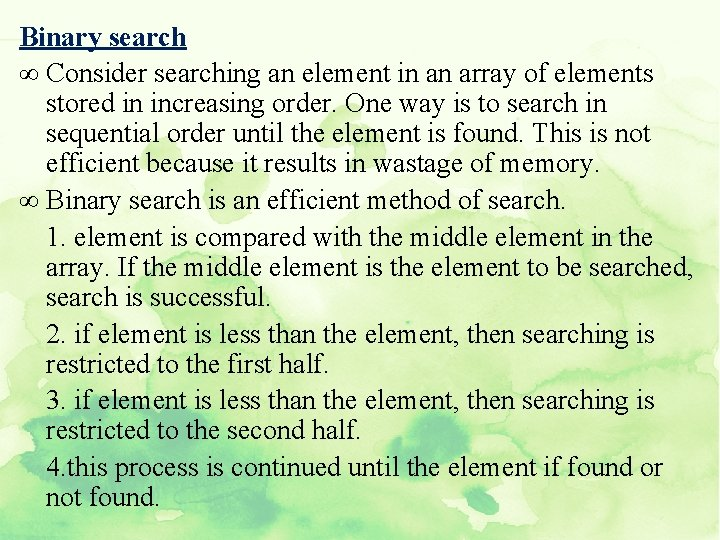Binary search ∞ Consider searching an element in an array of elements stored in increasing order. One way is to search in sequential order until the element is found. This is not efficient because it results in wastage of memory. ∞ Binary search is an efficient method of search. 1. element is compared with the middle element in the array. If the middle element is the element to be searched, search is successful. 2. if element is less than the element, then searching is restricted to the first half. 3. if element is less than the element, then searching is restricted to the second half. 4. this process is continued until the element if found or not found.1 2 3 4 5 6 7 8 9 0 1 2 3 4 5 6 7 8 Let the element to be searched is 2 Middle element is 5 and 2 is less than 5. hence first half is considered, which is 1 2 3 4 0 1 2 3 middle element is 2 and hence search is successful and element is found at position 1 1 2 3 4 5 6 7 8 9 0 1 2 3 4 5 6 Let the element to be searched is 10 6 7 8 9 5 6 8 9 7 8 7 10 is not found. 8 7 8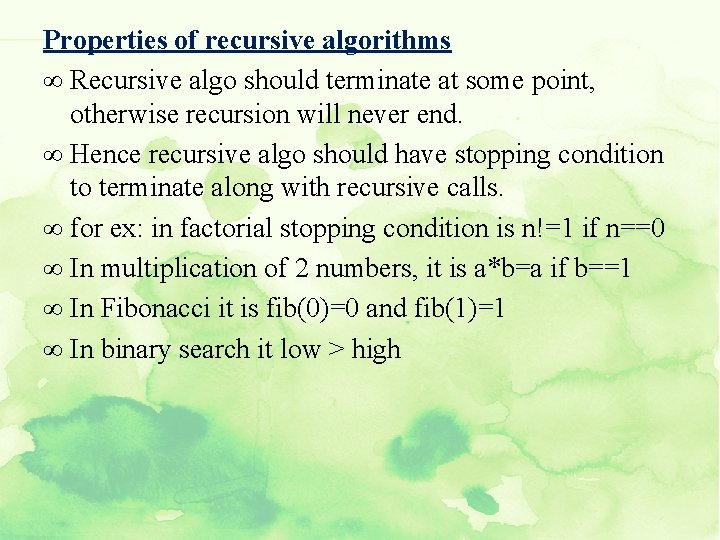Properties of recursive algorithms ∞ Recursive algo should terminate at some point, otherwise recursion will never end. ∞ Hence recursive algo should have stopping condition to terminate along with recursive calls. ∞ for ex: in factorial stopping condition is n!=1 if n==0 ∞ In multiplication of 2 numbers, it is a*b=a if b==1 ∞ In Fibonacci it is fib(0)=0 and fib(1)=1 ∞ In binary search it low > highFactorial in c Int fact(int n) { int x, y, res; if(n==0) return 1; else { x=n-1; y=fact(x); res=n*y; return res; } } Here y=fact(x), function gets called by itself each time with 1 less number than previous one until number gets zero.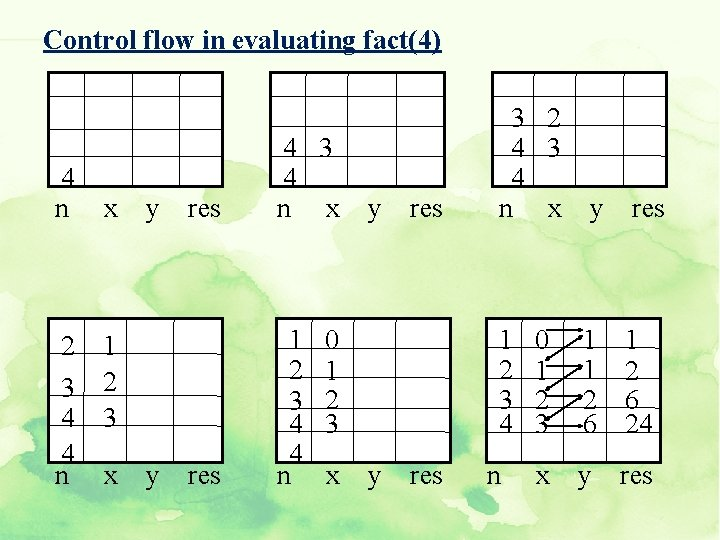Control flow in evaluating fact(4) 4 n x 2 1 3 2 4 3 4 n x y res 1 2 3 4 4 n y res 0 1 2 3 x y res 3 2 4 3 4 n x y res 1 2 3 4 0 1 2 3 1 1 2 6 x y res n 1 2 6 24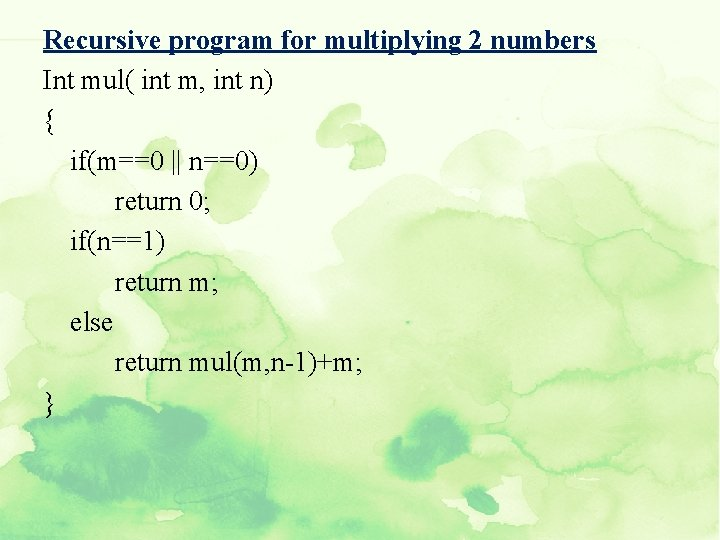Recursive program for multiplying 2 numbers Int mul( int m, int n) { if(m==0 || n==0) return 0; if(n==1) return m; else return mul(m, n-1)+m; }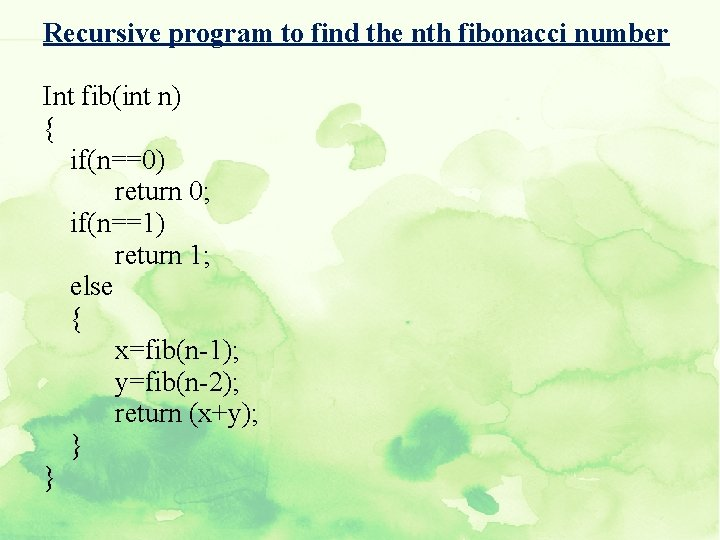Recursive program to find the nth fibonacci number Int fib(int n) { if(n==0) return 0; if(n==1) return 1; else { x=fib(n-1); y=fib(n-2); return (x+y); } }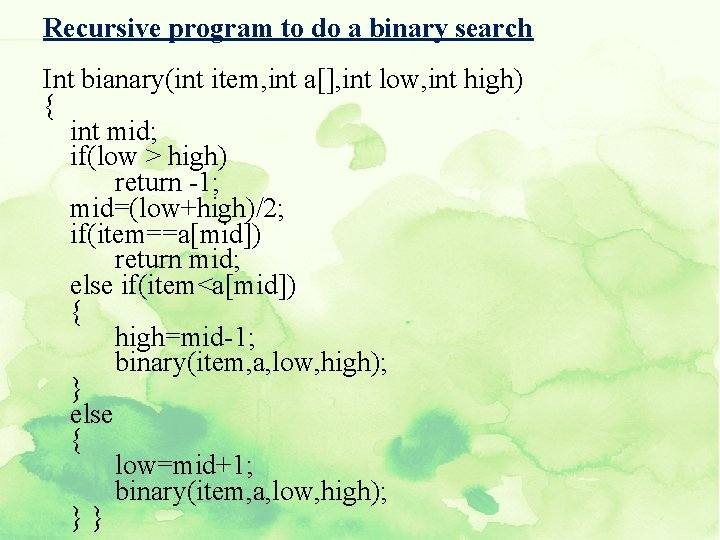Recursive program to do a binary search Int bianary(int item, int a[], int low, int high) { int mid; if(low > high) return -1; mid=(low+high)/2; if(item==a[mid]) return mid; else if(item<a[mid]) { high=mid-1; binary(item, a, low, high); } else { low=mid+1; binary(item, a, low, high); }}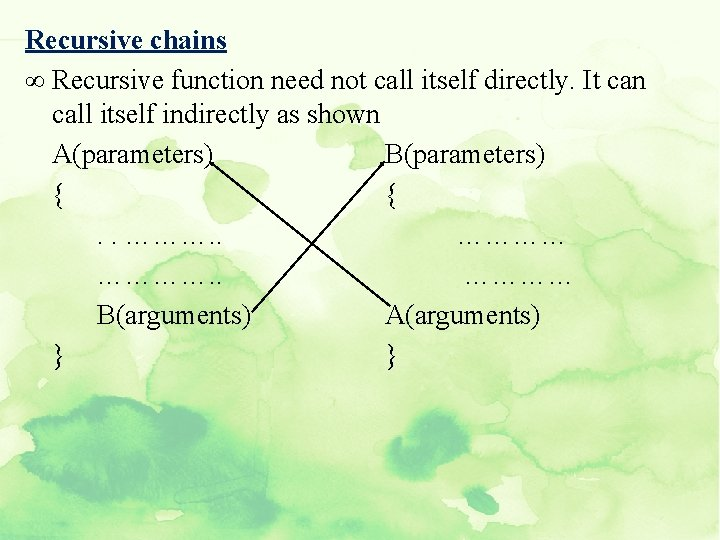Recursive chains ∞ Recursive function need not call itself directly. It can call itself indirectly as shown A(parameters) B(parameters) { {. . ………… B(arguments) A(arguments) } }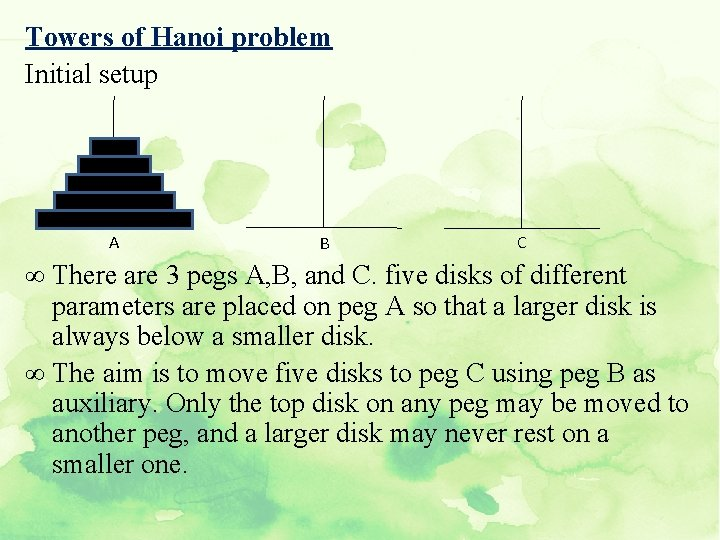Towers of Hanoi problem Initial setup A B C ∞ There are 3 pegs A, B, and C. five disks of different parameters are placed on peg A so that a larger disk is always below a smaller disk. ∞ The aim is to move five disks to peg C using peg B as auxiliary. Only the top disk on any peg may be moved to another peg, and a larger disk may never rest on a smaller one.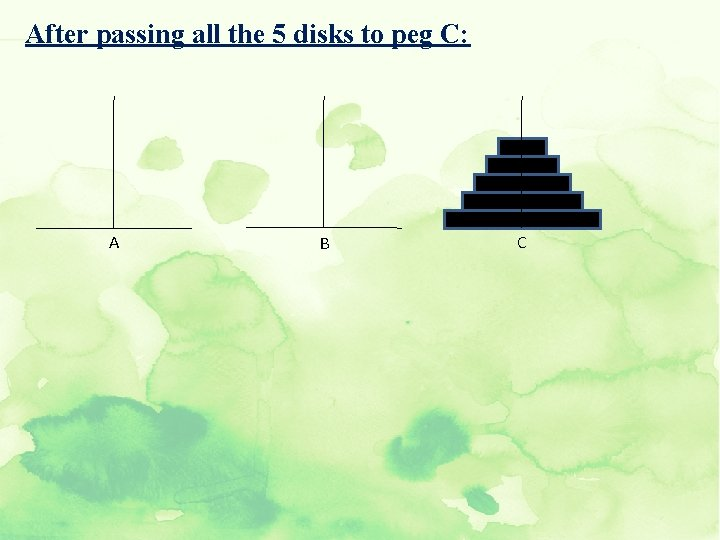After passing all the 5 disks to peg C: A B CLets consider the general case of n disks To move n disks from A to C using B as auxiliary 1. If n==1, move single disk from A to C. 2. Move the top n-1 disks from A to B using C as auxiliary. 3. Move the remaining disk from A to C. 4. Move the n-1 disks from B to C, using A as auxiliary. ∞ Here if n==1, step 1 will produce a correct solution. ∞ If n==2, we know that we already have a solution for n-1. i. e 1. so steps 2 and 4 can be performed. ∞ If n==3, we know that we have a solution for n-1. i. e 2. so steps 2 and 4 can be performed. ∞ In this way we have solutions for 1, 2, 3…. . upto any value. ∞ This clearly indicates the concept of recursion involved and hence this problem can be solved by recursion.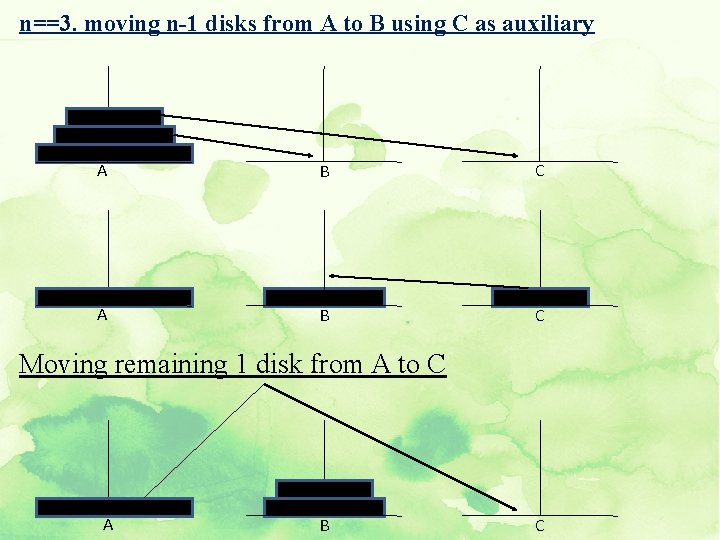n==3. moving n-1 disks from A to B using C as auxiliary A B C Moving remaining 1 disk from A to C A B C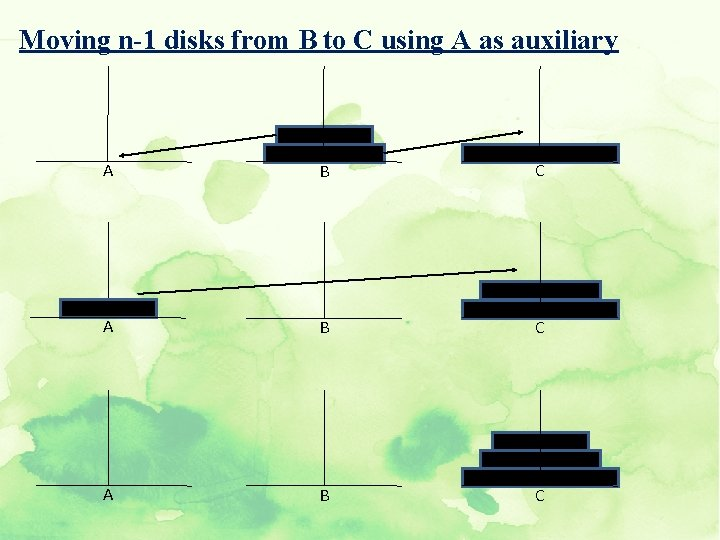Moving n-1 disks from B to C using A as auxiliary A B CC program for tower of hanoi problem void tower (int n, int source, int temp, int destination) { if(n==1) { printf(“move disk 1 from %c to %c”, source, destination); return; } /*moving n-1 disks from A to B using C as auxiliary*/ tower(n-1, source, destination, temp); printf(“move disk 1 from %c to %c”, source, destination); /*moving n-1 disks from A to B using C as auxiliary*/ tower((n-1), temp, source, destination); }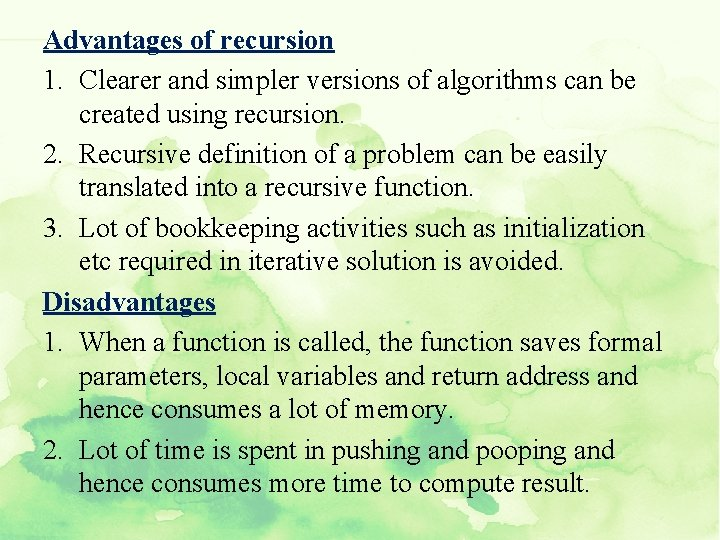Advantages of recursion 1. Clearer and simpler versions of algorithms can be created using recursion. 2. Recursive definition of a problem can be easily translated into a recursive function. 3. Lot of bookkeeping activities such as initialization etc required in iterative solution is avoided. Disadvantages 1. When a function is called, the function saves formal parameters, local variables and return address and hence consumes a lot of memory. 2. Lot of time is spent in pushing and pooping and hence consumes more time to compute result.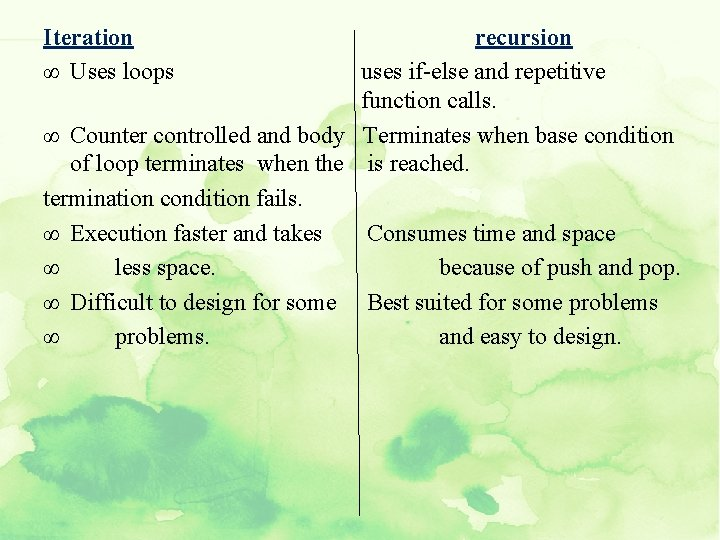Iteration ∞ Uses loops recursion uses if-else and repetitive function calls. ∞ Counter controlled and body Terminates when base condition of loop terminates when the is reached. termination condition fails. ∞ Execution faster and takes Consumes time and space ∞ less space. because of push and pop. ∞ Difficult to design for some Best suited for some problems ∞ problems. and easy to design.